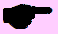# Mock Exam Paper B P2 (Project Maths) Higher Level

 Name Class Teacher SchoolPre-Leaving Certificate Examination, 2012

Mathematics

(Project Maths – Phase 1)

Paper 2

Higher Level

Time: 2 hours 30 minutes

 For examiner Question Mark 1 2 3 4 5 6 7 8 Total

300 marks

 Running total

Instructions

There are two sections in this examination paper.

Section A Concepts and Skills 150 Marks 6 questions

Section B Contexts and Applications 150 Marks 2 questions

Answer all eight questions as follows:

Questions 1 to 5 and

either Question 6A OR Question 6B

In Section B, answer Questions 7 and 8.

Write your answers in the spaces provided in this booklet. There is space for extra work at the back of the booklet. You may also ask the superintendent for more paper. Label any extra work clearly with the question number and part.

The superintendent will give you a copy of the booklet of Formulae and Tables. You must return it at the end of the examination. You are not allowed to bring your own copy into the examination.

Marks will be lost if all necessary work is not clearly shown.

Answers should include appropriate units of measurement, where relevant.

Answers should be given in simplest form, where relevant.

Write the make and model of your calculator(s) here:

Section A Concepts and Skills (150 marks)

Question 1 (25 marks)

(a) Three girls and four boys are to sit in a row of seven chairs. Find the probability that the three girls will be sitting beside each other?

(b) John and James play several games of pool against each other, each which may result in a win for John, a win for James or a draw. The probability that John wins any given game is 0.3 and the probability that James wins any given game is 0.2.

Calculate the probability that the players are level after:

(i) 2 games.

(ii) 3 games.

 page running

Question 2 (25 marks)

(a) The random variable X has a normal distribution with mean of 80 and a standard deviation of 8.58.

(i) Find

(ii)

(iii) Find the value of , correct to two deci...